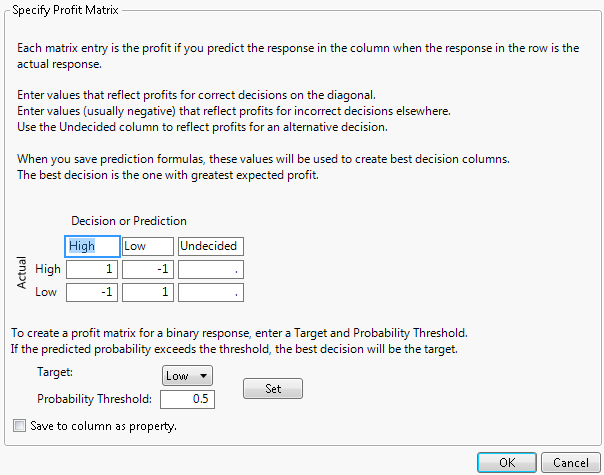Publication date: 11/10/2021

## Specify Profit Matrix

A profit matrix can be used with categorical responses. A profit matrix is used to assign costs to undesirable outcomes and profits to desirable outcomes.

Figure 4.13 Specify Profit Matrix WindowYou can assign profit and cost values to each combination of actual and predicted response categories. To specify the costs of classifying into an alternative category, enter values in the Undecided column. To save your assignments to the response column as a property, check Save to column as property. Leaving this option unchecked applies the Profit Matrix only to the current Partition report.

#### Probability Threshold Specification for Profit Matrix

When the response is binary, instead of entering weights into the profit matrix, you can specify a probability threshold in the Profit Matrix window. For more information about how values are calculated for the profit matrix, see Profit Matrix in Using JMP.

Target

The level whose probability is modeled.

Probability Threshold

A threshold for the probability of the target level. If the probability that an observation falls into the target level exceeds the probability threshold, the observation is classified into that level.

When you define costs using the Specify Profit Matrix option and then select Show Fit Details, a Decision Matrix report appears. See Decision Matrix Report.

When you specify a profit matrix and save the model prediction formula, the formula columns saved to the data table include the following:

Profit for <level>: For each level of the response, a column gives the expected profit for classifying each observation into that level.

Most Profitable Prediction for <column name>: For each observation, gives the level of the response with the highest expected profit.

Expected Profit for <column name>: For each observation, gives the expected profit for the classification defined by the Most Profitable Prediction column.

Actual Profit for <column name>: For each observation, gives the actual profit for classifying that observation into the level specified by the Most Profitable Prediction column.